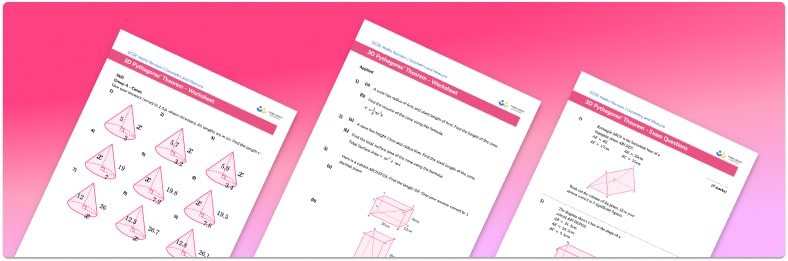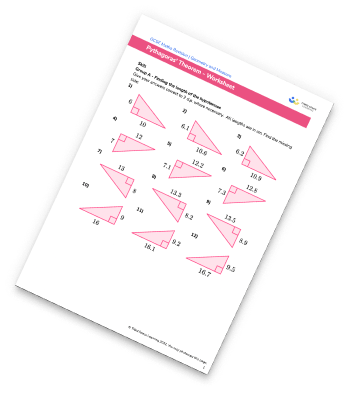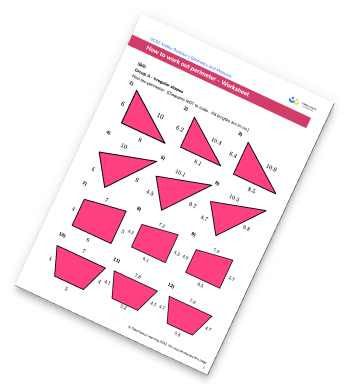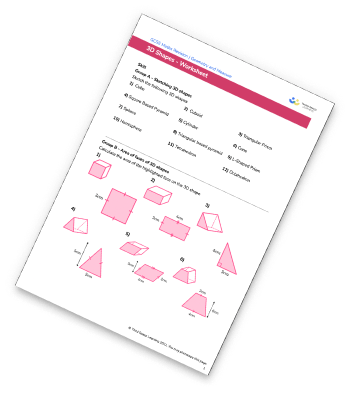# 3D Pythagoras Worksheet• This field is for validation purposes and should be left unchanged.

You can unsubscribe at any time (each email we send will contain an easy way to unsubscribe). To find out more about how we use your data, see our privacy policy.

• Section 1 of the 3d Pythagoras worksheet contains 27 skills-based 3d Pythagoras questions, in 3 groups to support differentiation
• Section 2 contains 5 applied 3d Pythagoras questions with a mix of worded problems and deeper problem solving questions
• Section 3 contains 3 foundation and higher level GCSE exam style questions on 3d Pythagoras
• Answers and a mark scheme for all 3d Pythagoras questions are provided
• Questions follow variation theory with plenty of opportunities for students to work independently at their own level
• All questions created by fully qualified expert secondary maths teachers
• Suitable for GCSE maths revision for AQA, OCR and Edexcel exam boards

### 3d Pythagoras at a glance

Once students are confident with using Pythagoras Theorem to find missing side lengths in right angle triangles in 2D, they can move on to solving problems using Pythagoras Theorem with 3D shapes.

A simple introductory example is looking at the longest length that can fit inside a cuboid-shaped box. If the base of the box is a rectangle abcd, the longest length inside that rectangle is the diagonal of the rectangle – e.g. the hypotenuse of the triangle abc. This hypotenuse then becomes a shorter side for another right angle triangle, with the height of the box as the other shorter side. The hypotenuse of this new triangle runs from one corner to the furthest opposite corner of the cuboid. As this is the longest length inside the box, so we would use another iteration of Pythagoras to work out this length.

Another common application is finding the perpendicular height of a square-based pyramid, possibly in an intermediate step before calculating its volume. If students are given the slant or slope height of the pyramid, they first need to apply Pythagoras Theorem to find the perpendicular height of one of the isosceles triangles on the side faces. A second right angle triangle is formed, using the length just calculated as its hypotenuse, and a line running to the midpoint of the square base as one of the shorter sides. A second iteration of Pythagoras theorem is then used to find the perpendicular height of the pyramid.

As square rooting often results in non-terminating decimals, students should give final answers to an appropriate number of decimal places, or leave their answer in surd form, as required.

Looking forward, students can then progress to additional geometry worksheets, for example a trigonometry worksheet or aFor more teaching and learning support on Geometry our GCSE maths lessons provide step by step support for all GCSE maths concepts.

## Related worksheets

Pythagoras WorksheetPlans And Elevations WorksheetPerimeter Worksheet3D Shapes Worksheets## Do you have KS4 students who need more focused attention to succeed at GCSE?There will be students in your class who require individual attention to help them succeed in their maths GCSEs. In a class of 30, it’s not always easy to provide.

Help your students feel confident with exam-style questions and the strategies they’ll need to answer them correctly with our dedicated GCSE maths revision programme.

Lessons are selected to provide support where each student needs it most, and specially-trained GCSE maths tutors adapt the pitch and pace of each lesson. This ensures a personalised revision programme that raises grades and boosts confidence.

Find out more